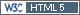## Usage of function CVfromCI (package PowerTOST) [R for BE/BA]

Dear participants of forum, I am relatively new to R and RStudio. And I really need your advise to clear some questions up which are important for my self-education and professional skills.

1. A BE study of Sotalol HCl 160 mg tablets (Mylan) and Betapace 160 mg tablets (Berlex Laboratories) with standard 2x2 cross-over design was described in one of the applications on FDA (application number 75-725). They planned to enroll 24 subjects. But in fact that study was conducted with 23 subjects. I would like to calculate CV from CI using data of that study. What data should I write for arguments "n" and "design"? If I input such data:

`CVfromCI(pe = 0.99, lower = 0.87, upper = 1.13, n = 23, design = "2x2", alpha = 0.05, robust=FALSE)`

RStudio gives me a result but with the following note:

```CVfromCI(pe = 0.99, lower = 0.87, upper = 1.13, n = 23, design = "2x2", alpha = 0.05, robust=FALSE) Unbalanced 2x2 design. n(i)= 12/11 assumed.  0.2617363```

Can I use this result for futher calculations of CVpooled by means of using PowerTOST or should I correct my data?

2. While calculating CV from CI with data from another study I`ve got the following result:

```CVfromCI(pe = 0.96, lower = 0.9075, upper = 1.0089, n = 24, design = "2x2", alpha = 0.05, robust=FALSE)  0.1071475 Warning message: sigma based on pe & lower CL more than 10% different than sigma based on pe & upper CL. Check input.```

My input is correct because numbers are taken from that application not from my head. Should I pay attention to this warning message and can I use this result for futher calculations of CVpooled by PowerTOST?

3. While calculating CV from CI is it prefered to use CI for log-transformed parameters (for example LCmax) or for non-transformed parameters(Cmax)?

Big thanks in advance.Ing. Helmut Schütz